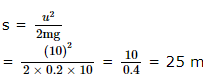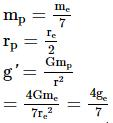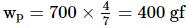Courses

# SRMJEEE Physics Mock Test - 3

## 35 Questions MCQ Test SRMJEEE Subject Wise & Full Length Mock Tests | SRMJEEE Physics Mock Test - 3

Description
This mock test of SRMJEEE Physics Mock Test - 3 for JEE helps you for every JEE entrance exam. This contains 35 Multiple Choice Questions for JEE SRMJEEE Physics Mock Test - 3 (mcq) to study with solutions a complete question bank. The solved questions answers in this SRMJEEE Physics Mock Test - 3 quiz give you a good mix of easy questions and tough questions. JEE students definitely take this SRMJEEE Physics Mock Test - 3 exercise for a better result in the exam. You can find other SRMJEEE Physics Mock Test - 3 extra questions, long questions & short questions for JEE on EduRev as well by searching above.
QUESTION: 1

Solution:
QUESTION: 2

Solution:
QUESTION: 3

### A coil of area 50cm2 is moved through a magnetic field of flux density 31 x 10−3 wb m−2 to 10−3 wb m−2 in 0.02s. The induced emf in the coil is

Solution:
QUESTION: 4

A motor cyclist moving with a velocity of 72 km/hour on a flat road takes a turn on the road at a point where the radius of curvature of the road is 20 meters. The acceleration due to gravity is 10 m/sec2. In order to avoid skidding, he must not bend with respect to the vertical plane by an angle greater than

Solution:
QUESTION: 5

An explosion blows a rock into three parts. Two parts go off at right angles to each other. These two are, 1 kg first part moving with a velocity of 12 ms− 1 and 2 kg second part moving with a velocity of 8 ms− 1. If the third part flies off with a velocity of 4 ms− 1, its mass would be:

Solution:
QUESTION: 6

The specific resistance of a wire is ρ, its volume is 3 m3 and its resistance is 3 ohms, then its length will be

Solution:
QUESTION: 7

A metal plate gets heated, when cathode rays against it, due to

Solution:
QUESTION: 8

For a given material, the Young's modulus is 2.4 times that of rigidity modulus. Its Poisson's ratio is

Solution:
QUESTION: 9

A common emitter amplifier has a voltage gain of 50, an input impedance of 100 ohm, and an output impedance of 200 ohm. The power gain of the amplifier will be:

Solution:
QUESTION: 10

When two capacitors of capacities 3 μF and 6 μF are connected in series and connected to 120V, the potential difference across 3 μF is

Solution:
QUESTION: 11

On a rough horizontal surface, a body of mass 2 kg is given a velocity of 10 m/s. If the coefficient of friction is 0.2 and g = 10 m/s2, the body will stop after covering a distance of

Solution:QUESTION: 12

In a room where the temprature is 30°C, a body cods from 61°C to 59°C in 4 minutes. The time (in min.) taken by the body to cool from 51°C to 49°C will be

Solution:
QUESTION: 13

The r.m.s. speed of the molecules of a gas in a vessel is 400 ms⁻1. If half of the gas leaks out, at constant temperature, the r.m.s. speed of the remaining molecules will be

Solution:
QUESTION: 14

Which of the following is NOT an illustration of Newton's third law ?

Solution:
QUESTION: 15

An ammeter and a voltmeter of resistance R are connected in series to an electric cell of negligible internal resistance. Thier readings are A and V respectively. If another resistance R is connected in parallel with the voltmeter.

Solution:
QUESTION: 16

The incorrect statement regarding the lines of force of the magnetic field B is

Solution:
QUESTION: 17

What is the weight of a 700 g of body on a planet whose is 1/7 th of that of earth and radius is 1/2 times of earth ?

Solution:QUESTION: 18

A beaker containing a liquid of density (ρ) moves up with an acceleration (a). The pressure due to liquid at a depth (h) below the surface of the liquid is

Solution:
QUESTION: 19

An aeroplane is moving with horizontal velocity u at height h. The velocity of a packet dropped from it on the earth's surface will be (g is acceleration due to gravity)

Solution:
QUESTION: 20

A ball is rolled off along the edge of table with velocity 4 m/s. It hits the ground after time 0.4 s. Which one of the following is wrong? (g = 10 m/s2)

Solution:
QUESTION: 21

A boy whose mass is 50 kg stands on a spring balance inside a lift. The lift starts to ascent with an acceleration of 2ms⁻2. The reading of the machine or balance (g = 10 ms⁻2) is

Solution:
QUESTION: 22

If a spring extends by x on loading, then the energy stored by the spring is (if T is tension in the spring and k is spring constant)

Solution:
QUESTION: 23

Two points particles with masses m₁ and m₂ are thrown at angles θ₁ and θ₂ with the horizontal with speed ν₁ and ν₂ respectively. T,H and R represent the total time of flight, the maximum height attained and the horizontal range covered respectively. If ν₁ sin θ₁ = ν₂ sin θ₂, then for both the paricles

Solution:
QUESTION: 24

The total weight of a piece of wood is 6 kg. In the floating state in water its 1/3 part remains inside the water. On this floating solid, what maximum weight is to be put such that the whole of the piece of wood is to be drowned in the ratio?

Solution:
QUESTION: 25

The distance between eye lens and cross wire in Ramsden eye piece which has a field lens of focal length 1.2 cm is .......cm.

Solution:
QUESTION: 26

A stone is tied up to the end of a string and whirled in a vertical circle. The tension in the string is T1 when the stone is at the highest point and T2 when it is at the lowest point. Then

Solution:
QUESTION: 27

Newton per square metre is the unit of

Solution:
QUESTION: 28

The surface tension of soap solution is 25x10⁻3Nm⁻1. The excess pressure inside a soap bubble of diameter 1 cm is

Solution:
QUESTION: 29

A current i passes through a wire of length l, radius of cross-section r and density ρ. The rate of heat generation is

Solution:
QUESTION: 30

It is difficult to cook rice in an open vessel by boiling it at high altitudes because of

Solution:
QUESTION: 31

The penetration of light into the region of geometrical shadow is called

Solution:
QUESTION: 32

For a perfect gas, if α and β are volume and pressure coefficients of expansion.

Solution:
QUESTION: 33

A body of mass 10 kg at rest is acted upon simultaneously by two forces 4 N and 3 N at right angles to each to each other. The kinetic energy of the body at the end of 10 sec is

Solution:
QUESTION: 34

Which of the following is not the unit of time?

Solution:
QUESTION: 35

A source and a listener are moving towards each other with a speed 1/10th of the speed of sound. if the frequency of the note emitted by the source is f, the frequency of the note heard by the listner will be nearly

Solution: